# 6th Grade Math Triangle Interior Angle Sum

Page

Video:

Hypothosis, Demo of the why, and one practice probelm

https://www.mathsisfun.com/proof180deg.html

Practice finding the missing angle here:

http://www.mathopolis.com/questions/q.php?id=732&site=1&ref=2f70726f6f663138306465672e68746d6c&title=547269616e676c657320436f6e7461696e203138302044656772656573&qs=732_1518_733_1519_2139_2140_3938_3939_2141_2142

# Triangles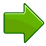A triangle has three sides and three angles SIDES can be measured in inches, feet, centimeters, etc.The three angles always add to 180° ANGLES are ALWAYS measured in DEGREES °

## Equilateral, Isosceles and Scalene, what are the SIDE LENGTHS?

There are three special names given to triangles depending on its sides.

These names that tell you how many sides (or angles) are EQUAL.

There can be 32 or no equal sides/angles: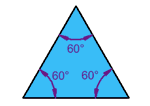### Equilateral Triangle (EQUAL)

Three equal sides
Three equal angles, always 60°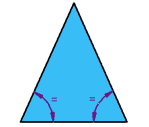### Isosceles Triangle (Interesting)

Two equal sides
Two equal anglesNo equal sides
No equal angles

## What Type of Angle?

Triangles can also have names that tell you what type of angle is inside: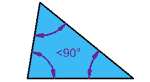### Acute Triangle  (Cute and Small)

All angles are less than 90°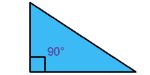### Right Triangle  (Just Right!)

Has a right angle (90°)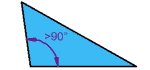### Obtuse Triangle  (Oh wow that’s big)

Has an angle more than 90°

## Combining the Names

Sometimes a triangle will have two names, for example: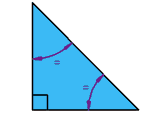### Right Isosceles Triangle

Has a right angle (90°), and also two equal sides and two equal angles.

Can you guess the measurement of the two equal angles?